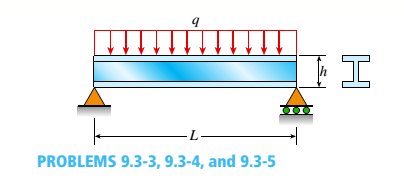# A wide-flange beam (W 12 x 35) supports a uniform load on a simple span of length L = 14 ft (see figure). Calculate the maximum deflection δ max at the midpoint and the angles of rotation Sat the supports if q = 1.8 kips/ft and E = 30 × 10 6 psi. (Use the formulas of Example 9-1.)### Mechanics of Materials (MindTap Co...

9th Edition
Barry J. Goodno + 1 other
Publisher: Cengage Learning
ISBN: 9781337093347### Mechanics of Materials (MindTap Co...

9th Edition
Barry J. Goodno + 1 other
Publisher: Cengage Learning
ISBN: 9781337093347
Chapter 9, Problem 9.3.3P
Textbook Problem
5 views

## A wide-flange beam (W 12 x 35) supports a uniform load on a simple span of length L = 14 ft (see figure).Calculate the maximum deflection δ max at the midpoint and the angles of rotation Sat the supports if q = 1.8 kips/ft and E = 30 × 106 psi. (Use the formulas of Example 9-1.)To determine

The maximum deflection δmax and the angle of rotation θ .

### Explanation of Solution

Given information:

L=14ftq=1.8kips/ftE=30×106psiW12×35

Formula used:

δmax=5qL4384EI

θ=θA=θB=qL324EI

Calculation:

Let us first convert the units;

L=14ft=168in and q=1.8k/ft=150lb/in ;

I=285in4

We know the formula for the maximum deflection, i.e

### Still sussing out bartleby?

Check out a sample textbook solution.

See a sample solution

#### The Solution to Your Study Problems

Bartleby provides explanations to thousands of textbook problems written by our experts, many with advanced degrees!

Get Started

Find more solutions based on key concepts
How can a tap be aligned when threading a hole on the lathe?

Precision Machining Technology (MindTap Course List)

List and describe the three major steps in executing the project plan.

Principles of Information Security (MindTap Course List)

What is structural independence, and why is it important?

Database Systems: Design, Implementation, & Management

The cash flow given in Problem 20.18 is to be replaced by an equivalent cash flow with equal amounts (X) at the...

Engineering Fundamentals: An Introduction to Engineering (MindTap Course List)

The force R is the resultant of P and 0. Determine Q and the angle .

International Edition---engineering Mechanics: Statics, 4th Edition

What is an RFP, and how does it differ from an RFQ?

Systems Analysis and Design (Shelly Cashman Series) (MindTap Course List)

List at least three hardware devices listed in the System Report or in the Device Manager.

Enhanced Discovering Computers 2017 (Shelly Cashman Series) (MindTap Course List)

Describe how a person should safely lift a heavy object.

Welding: Principles and Applications (MindTap Course List)

If your motherboard supports ECC DDR3 memory, can you substitute non-ECC DDR3 memory?

A+ Guide to Hardware (Standalone Book) (MindTap Course List)